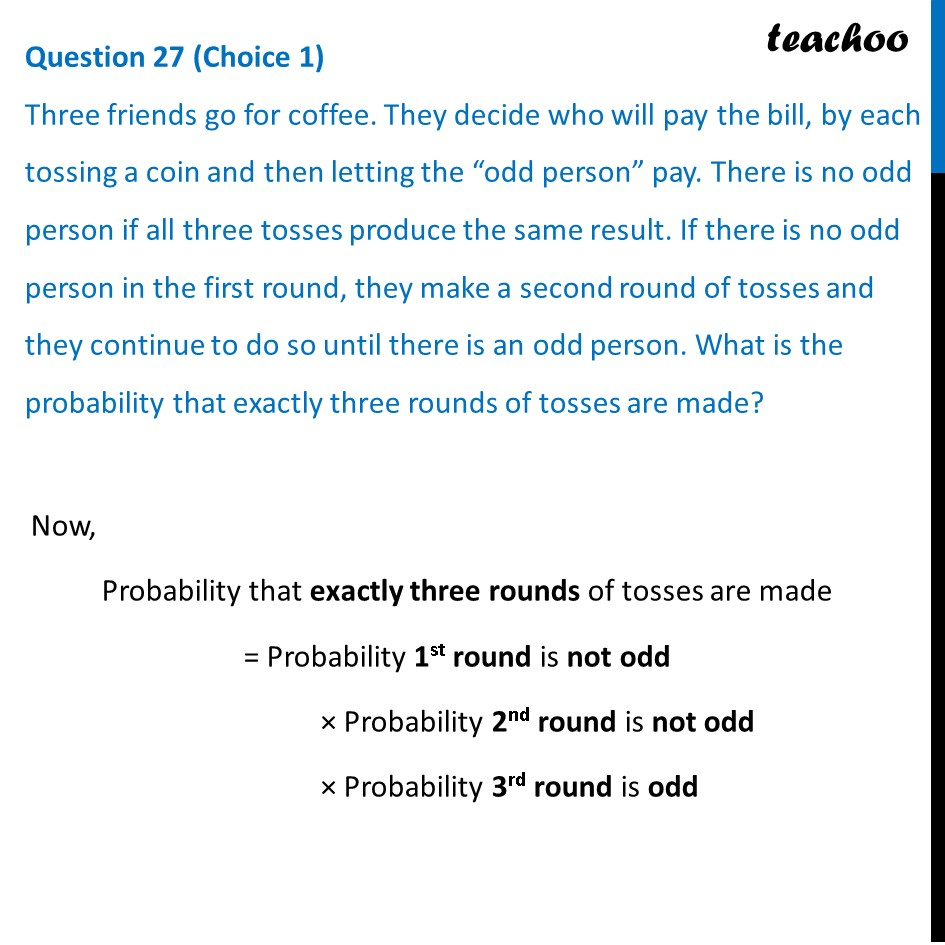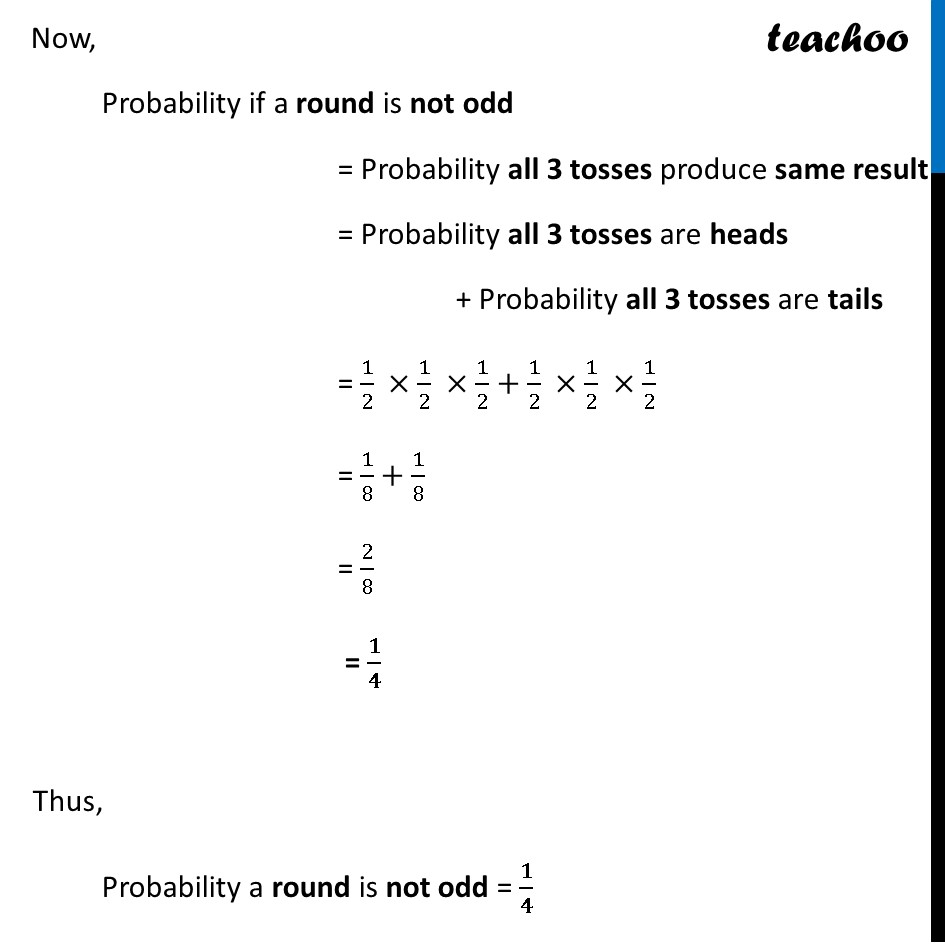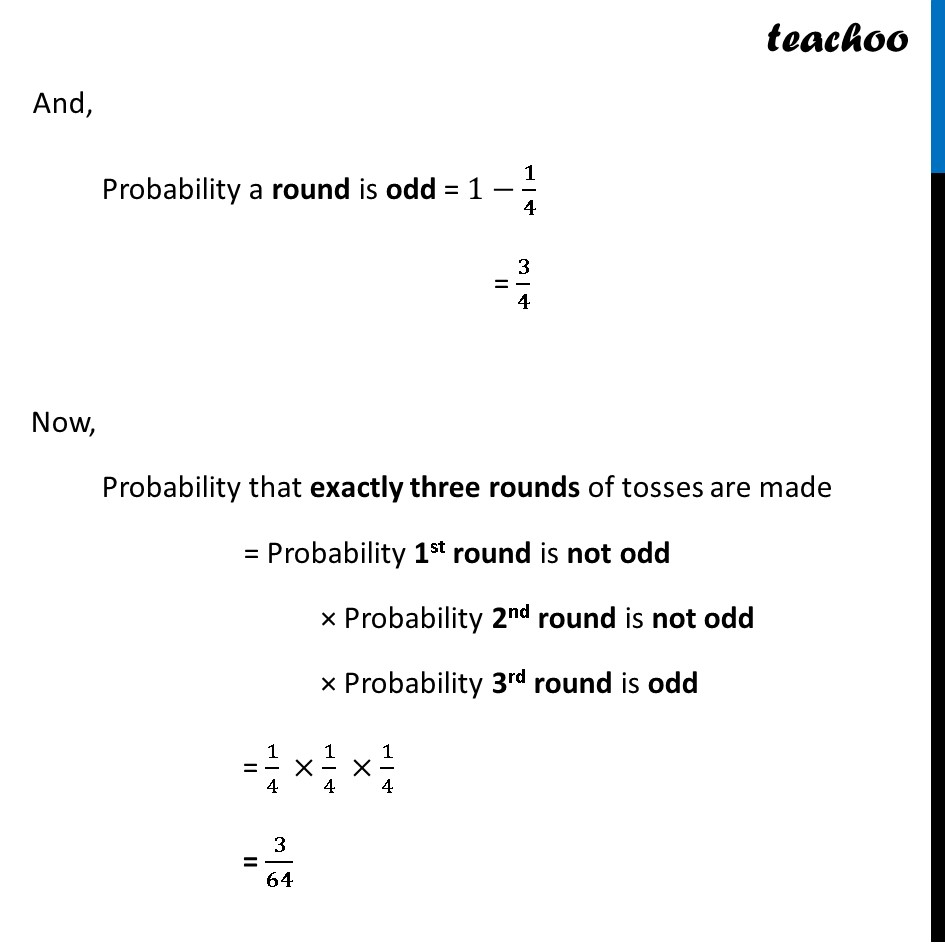CBSE Class 12 Sample Paper for 2023 Boards

Class 12
Solutions of Sample Papers and Past Year Papers - for Class 12 Boards

## Three friends go for coffee. They decide who will pay the bill, by each tossing a coin and then letting the “odd person” pay. There is no odd person if all three tosses produce the same result. If there is no odd person in the first round, they make a second round of tosses and they continue to do so until there is an odd person. What is the probability that exactly three rounds of tosses are made?Learn in your speed, with individual attention - Teachoo Maths 1-on-1 Class

### Transcript

Question 27 (Choice 1) Three friends go for coffee. They decide who will pay the bill, by each tossing a coin and then letting the “odd person” pay. There is no odd person if all three tosses produce the same result. If there is no odd person in the first round, they make a second round of tosses and they continue to do so until there is an odd person. What is the probability that exactly three rounds of tosses are made? Now, Probability that exactly three rounds of tosses are made = Probability 1st round is not odd × Probability 2nd round is not odd × Probability 3rd round is odd Now, Probability if a round is not odd = Probability all 3 tosses produce same result = Probability all 3 tosses are heads + Probability all 3 tosses are tails = 1/2 ×1/2 ×1/2+1/2 ×1/2 ×1/2 = 1/8+1/8 = 2/8 = 𝟏/𝟒 Thus, Probability a round is not odd = 𝟏/𝟒 And, Probability a round is odd = 1−𝟏/𝟒 = 𝟑/𝟒 Now, Probability that exactly three rounds of tosses are made = Probability 1st round is not odd × Probability 2nd round is not odd × Probability 3rd round is odd = 1/4 ×1/4 ×1/4 = 𝟑/𝟔𝟒